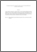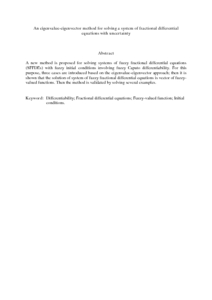# An eigenvalue-eigenvector method for solving a system of fractional differential equations with uncertainty

## Citation

Shahriyar, M. R. Balooch and Ismail, Fudziah and Aghabeigi, S. and Ahmadian, Ali and Salahshour, S. (2013) An eigenvalue-eigenvector method for solving a system of fractional differential equations with uncertainty. Mathematical Problems in Engineering, 2013. art. no. 579761. pp. 1-11. ISSN 1024-123X

## Abstract

A new method is proposed for solving systems of fuzzy fractional differential equations (SFFDEs) with fuzzy initial conditions involving fuzzy Caputo differentiability. For this purpose, three cases are introduced based on the eigenvalue-eigenvector approach; then it is shown that the solution of system of fuzzy fractional differential equations is vector of fuzzy-valued functions. Then the method is validated by solving several examples.Preview
PDF (Abstract)
An eigenvalue.pdfView Item